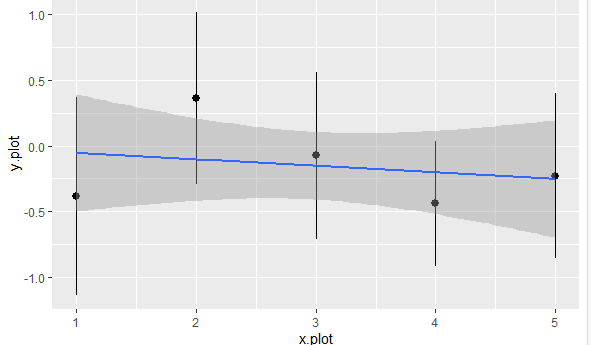2 views

I'm trying hard to add a regression line on a ggplot. I first tried with abline but I didn't manage to make it work. Then I tried this...

data = data.frame(x.plot=rep(seq(1,5),10),y.plot=rnorm(50))

ggplot(data,aes(x.plot,y.plot))+stat_summary(fun.data=mean_cl_normal) +

geom_smooth(method='lm',formula=data\$y.plot~data\$x.plot)

But it is not working either.

by

To plot a regression line on the plot, you can do the following:

data = data.frame(x.plot=rep(seq(1,5),10),y.plot=rnorm(50))

ggplot(data,aes(x.plot,y.plot))+stat_summary(fun.data=mean_cl_normal) +

geom_smooth(method='lm',formula=y~x)

Output:When you provide your own formula, you have to use the arguments that correspond to the arguments provided in the ggplot function.
If you are using the same x and y values that you provided in the ggplot function then you don’t need to use the formula inside the geom_smooth function.i.e.,
ggplot(data,aes(x.plot,y.plot))+stat_summary(fun.data=mean_cl_normal) +
geom_smooth(method='lm')